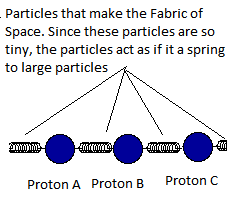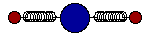# Trying to make sense of the Fabric Of Space (The field that consists of the smallest particle-like phenomenon )

#### kai99

So I have been trying to make sense of what space-time is from a practical point view. I thought of sharing with you some thoughts that came to my mind.

1). The fabric of space must be made out of the smallest wave-like phenomenon. Since this is so tiny, think of it as the smallest particle. These particles (or let's call it a field), fill the entire universe.
2). When we place a heavy particle in this field, the particle would pull this field/the fabric of space (that acts as if it a spring) in every direction towards the particle and that's based on the mass of the particle that we place in this field. This pulling force that exerts on the spring, would depend on the mass of the particle. So let's say we place three protons next to each other in this field. If so, these three protons would pull the spring towards them with an equal force (since the mass of the protons is the same), therefore, protons can’t get close to each other. Similarly, two electrons would also pull the fabric of space towards them with an equal force, even electrons can’t get closer each other.3). Now, let's say we place a proton and an electron close to each other. Since the mass of the proton is high, compares to the mass of the electron, the proton would pull the electron closer since its pulling power on the Fabric of Space is much larger (note that this pulling power depends on the mass of the particle). Since a proton pulls an electron closer, we call protons as positively charge and the electron as negatively charge (this is what we call as a charge).(Imagine, proton (the blue ball) and the electron (the small red balls) pull the Fabric of Space towards each other, but since the proton's mass is larger than the electron, its pulling power on the Fabric of Space is larger, so the proton can pull these electrons towards it. More, protons means, there's more force on the Fabric of Space, so it is subject to a larger contraction (imagine, this spring getting squeezed), compares to a contraction of a single proton.

The Four Forces:

The mass of the particle and/or object, would always distort the fabric of space however and how far it can affect the fabric/field would depend on the mass of the particle. For instance, since the size of the proton is small, its effect on the Fabric of Space span only a tiny distance and when it comes to quarks, it’s even smaller.

Strong Force:

Since the mass of the neutron is larger than the proton, the neutron pulls the Fabric of Space with a great force than a proton, therefore, it pulls the Proton towards it. Since the masses of these particles (neutrons and protons) is larger than the electrons, their pulling power is larger, so we see this as the strong force (which we see inside a nucleus).

Weak Force:

The quakes that make the protons and the neutrons are smaller than protons and the neutrons, and their mass is also small. This means their pulling power is also small. So, we see it as the weak force.

Electromagnetic Force:

Since the mass of the proton is larger than an electron, it pulls the Fabric of Space towards it with a greater force, so for instance, it pulls the electron in a hydrogen atom. We call this the electromagnetic force.
In a helium atom, this force is large, since its nucleus is made up of a neutron and proton that has almost identical masses.

Gravity:

When we talk about Gravity, we talk about planets and other massive objects which are made up of a massive amount of atoms. Since we are referring to a planet, on this occasion atoms as a whole pull the Fabric of Space towards the planet, so the Fabric of Space or this field that consists of the smallest particle-like phenomenon contracts.

Since the size of planets is massive compare to protons, neutrons, and electrons, planets can affect the Fabric of Space over larger distances. And that's because its pulling power on the Fabric of Space is so high. This pulling force on the Fabric of Space is high on the surface of the planet (Therefore, the amount of the contraction on the surface is high. On the other hand this increases the density of the smallest particle-like phenomenon), compares to the pulling force exert on the Fabric of Space at a distance from the planet (therefore, the amount of contraction gets smaller at a distant, this deceases the density of the smallest particle-like phenomenon). When this pulling force becomes weak, the gravity becomes weak (We know that this strength is inversely proportional to the square of the distance from the center of the Earth. So, the effect rapidly declines).

Bending of Light around a body:

Since large objects contract the Fabric of space (or this field consists of the smallest particle-like phenomenon) over larger distances, the density of the smallest particle-like phenomenon increase on the surface of the planet (the Fabric of Space contracts), and this density decreases as it moves away from the planet (the Fabric of Space contracts but much less compares to the surface). In other words, massive objects distort the Fabric of Space (Einstein called this as curvature of spacetime) or the field that consists of particle-like phenomenon. Therefore, this curvature changes the path of light.

Light Energy Loss:

“This is fundamentally what happens to light, as it travels through an expanding universe. The universe as a whole is expanding, meaning that the space between many galaxies is increasing. As light travels away from a galaxy, the Universe is continually expanding, meaning that the distance the light needs to travel is continually increasing as well. As space stretches out underneath a beam of light, its wavelength increases, and its energy decreases”
Source: https://www.forbes.com/sites/jillianscudder/2017/04/28/astroquizzical-light-energy-loss/?sh=22cdf7815768

Alternative explanation

The reason why wavelength is increasing is that the tension of the fabric of space between the particles that fabric is made up of reduces (this is because galaxies/planets are not affecting the fabric of space to contract that much when we go towards the edge of the space). Therefore, the distance between these particles increases (the particle-like phenomenon that the fabric of space is made up of), this increases the wavelength of a photon. When wavelength increases, its frequency decreases, therefore, photons' perceived color would change. This can be identified as the reason behind redshift.

(There’s no such a thing called space-time. It’s a mistake).

However, the fabric of space does experience a contraction or expansion depends on the particles/objects in a given location in space. The more the mass, the planet/object or a particle has, the pulling power increases, this is what causes the fabric to contract (or the particle-like phenomenon that the fabric of space is made up of